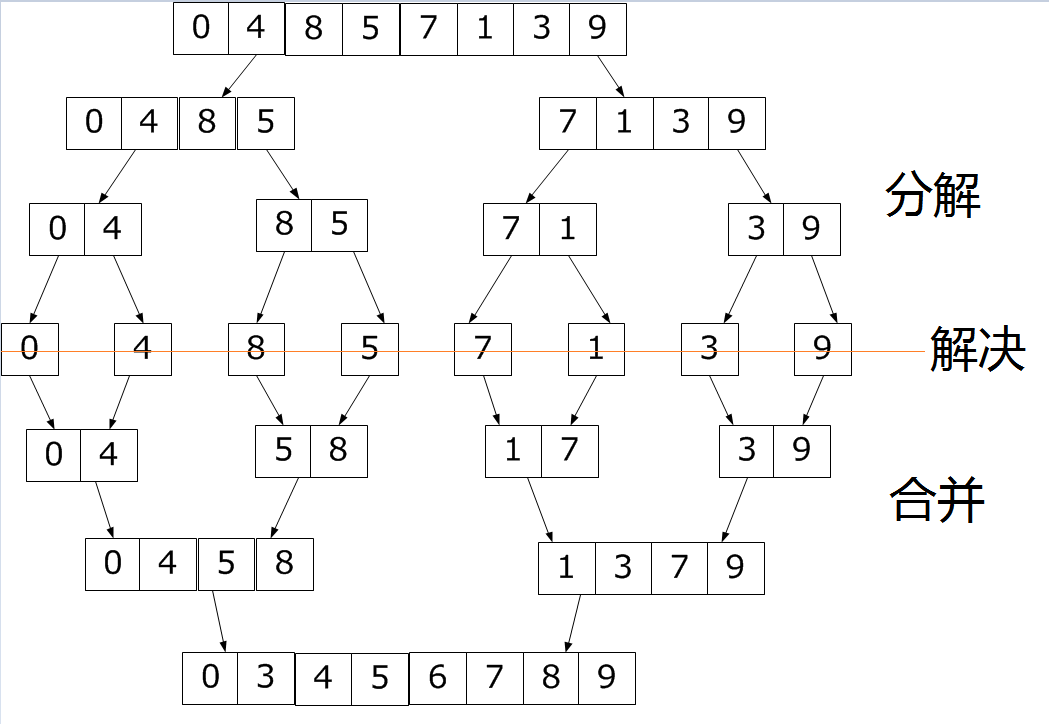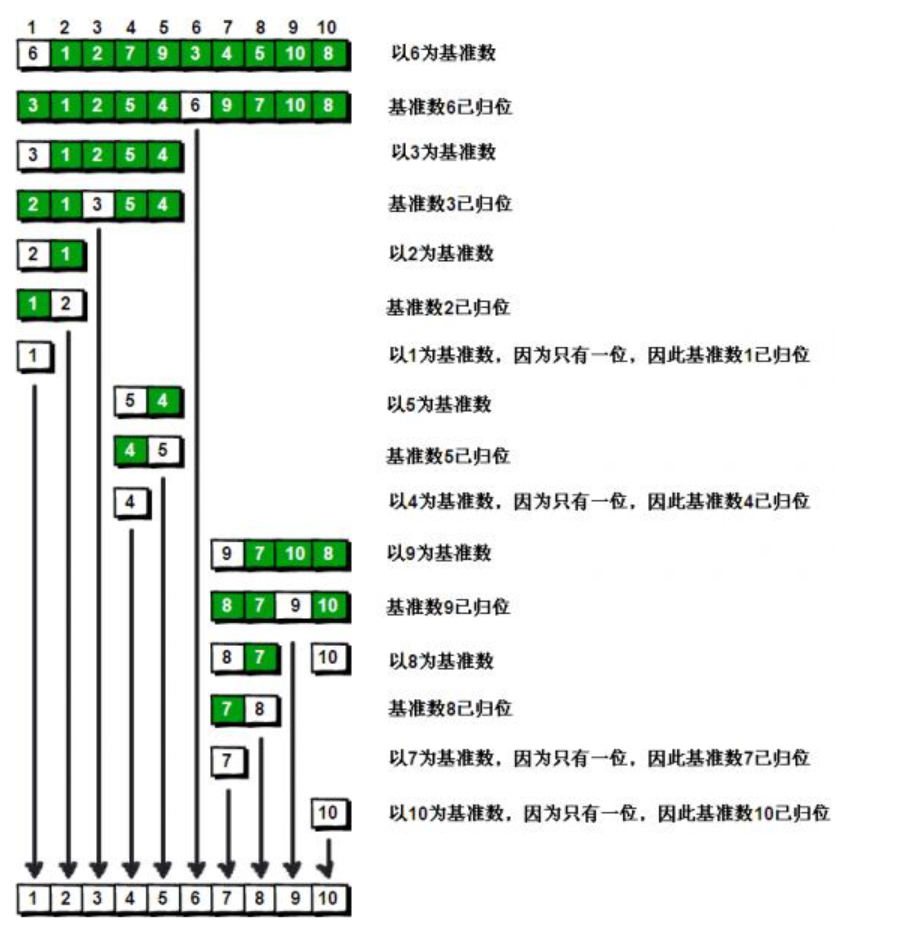# 排序算法——归并排序与快速排序package SORT;

public class MergeSort {

public static void mergeSort(int[] arr) {
int[] temp = new int[arr.length];
mergeSort(arr, 0, arr.length - 1, temp);
}

public static void mergeSort(int[] arr, int left, int right, int[] temp) {
//参数分别为 待排序数组，左指针，有指针，辅助数组
//因为使用了递归，所以我们必须规定递归条件否则将进行无线循环
while (left < right) {
//将数组进行分割
int mid = (left + right) / 2;
//对左子数组继续进行归并排序
mergeSort(arr, left, mid, temp);
//对右子数组继续进行归并排序
mergeSort(arr, mid + 1, right, temp);
//将数组进行合并
Merge(arr, left, mid, right, temp);
}

}
//合并函数
public static void Merge(int[] arr, int left, int mid, int right, int[] temp) {
int i = left;
int j = mid + 1;
//t为辅助数组的索引
int t = 0;
while (i <= mid && j <= right) {
//当二者都没有到达最后一位时，进行比较并向辅助数组复制
if (arr[i] < arr[j]) {
temp[t++] = arr[i++];
} else {
temp[t++] = arr[j++];
}
}
//当其中一个数组复制完毕后，将另一个数组内的数组全部复制进辅助数组
while (i <= mid) {
temp[t++] = arr[i++];
}
while (j <= right) {
temp[t++] = arr[j++];
}
t = 0;
//将辅助数组内已经排好的数据全部复制进原数组，排序完成
while (left <= right) {
arr[left++] = temp[t++];
}

}

}

• 快速排序是原地排序（只需要非常小的一个辅助栈）
• 快速排序时间消耗，长度为N的数组排序时间与NlgN成正比package SORT;

public class QuickSort {
public static void quickSort(int[] arr) {
// 对函数进行封装
quickSor(arr, 0, arr.length - 1);
}

public static void quickSor(int[] arr, int left, int right) {
if (left >= right) {
return;
}
int i = left;// 左哨兵
int j = right;// 右哨兵
int index = arr[i];// 基准数
int t = 0;

while (i < j) {
//当右侧数据大于基准数时，右指针向左扫描
while (arr[j] > index) {
j--;
}
//当左侧数据小于基准数时，左指针右左扫描
while (arr[i] < index) {
i++;
}
//当二者都停下时，交换数据
if (i < j) {
t = arr[i];
arr[i] = arr[j];
arr[j] = t;
}
//当整个过程完成时，将基准数和分界处的数据互换
arr[left] = arr[i];
arr[i] = index;
//递归处理
quickSor(arr, left, i - 1);
quickSor(arr, i + 1, right);
}
}
}


if (left + M >= right) {
//插入排序代码，具体操作不进行实现
sort(arr,left,right);
return;
}

01-27743
08-148103-213708
10-252199
10-13167
05-221万+
10-06448
06-04129
04-027573
06-06156